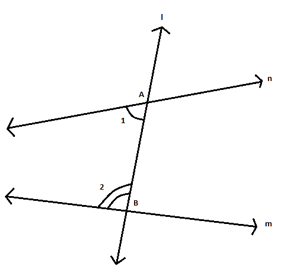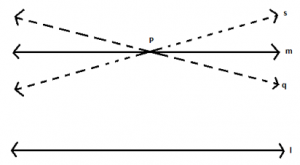# Euclid Geometry

Euclid Geometry: Euclid, a teacher of mathematics in Alexandria in Egypt, gave us a remarkable idea regarding the basics of geometry, through his book called ‘Elements’. His axioms and postulates are studied until now for a better understanding of the subject. In this article, you will be concentrating on the equivalent version of his 5th postulate given by John Playfair, a Scottish mathematician in 1729.

Before understanding the equivalent version of Euclid’s fifth postulate, go through the fifth postulate first.

## Fifth postulate of Euclid geometry

If a straight line falling on two straight lines makes the interior angles on the same side of it taken together less than two right angles, then the two straight lines, if produced indefinitely, meet on that side on which the sum of angles is less than two right angles.In the given diagram, the sum of angle 1 and angle 2 is less than 180°, so lines n and m will meet on the side of angle 1 and angle 2.

Now let us focus on the equivalent version of Euclid’s fifth postulate given by John Playfair. As per him:

“In a plane, given a line and a point not on it, at most one line parallel to the given line can be drawn through the point”.

In simple words, the above statement can be written as:

‘For every line l and for every point P not lying on l, there exists a unique line m passing through P and parallel to l’.In the above figure, consider line l and a point P not lying on l. Now, we know that an infinite number of lines can pass through a given point. Thus, infinite lines can pass through point P. But, are all the lines parallel to line l or are some of them parallel to line l or are none of them parallel to line l? According to Playfair there is only line passing through P which will be parallel to the line l.

Draw any line passing through point P, say line s. If you draw a transversal cutting lines s and l and passing through point P, you can see that on one of the sides, the sum of co-interior angles will be less than 180° thus both the lines will meet in that direction. There is only one possibility, i.e. line m, in which the sum of the co-interior angles if exactly 180° on both sides. Hence, in this case, both lines will never meet.

Thus, we can observe that this axiom by itself is not inevitably (or logically) equivalent to the Euclidean parallel postulate as there are geometries under which one is true, and the other is not. Still, each of these can be used to prove the other in the presence of the remaining axioms which give Euclidean geometry; therefore, they are said to be equivalent in terms of absolute geometry.